# Alperin conjecture

Jump to: navigation, search

Alperin weight conjecture

Modular representation theory of finite groups is the study of representations of groups over fields of finite characteristic (cf. also Finite group, representation of a; Finite group; Field). This theory was first developed by R. Brauer, who was motivated largely by a wish to obtain information about complex characters of finite groups (cf. Character of a group). One of the central themes of Brauer's work was that many representation-theoretic invariants of finite groups should be "locally" determined. Whenis a prime number, a-local subgroup of a finite group is the normalizer (cf. also Normalizer of a subset) of a non-trivial-subgroup of that group. An important feature of Alperin's weight conjecture is that it makes a precise prediction as to how a fundamental representation-theoretic invariant should be-locally controlled.

Let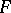be an algebraically closed field of prime characteristic, and letbe a finite group. Then the group algebra has a unique decomposition in the form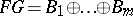, where the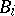's are mutually annihilating indecomposable two-sided ideals (cf. Ideal). The's are known as blocks of(cf. also Block). Let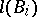denote the number of isomorphism types of simple-modules, and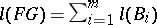. It is sometimes the case thatis isomorphic to a full matrix algebra over. In that case,is a block of defect zero of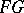. Such blocks have special significance; they are in bijection with the isomorphism types of projective simple-modules. Blocks other than blocks of defect zero are said to have positive defect.

A weight ofis a pair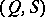, whereis a (possibly trivial)-subgroup (cf. also-group) ofandis a projective simple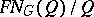-module. Weightsand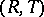are deemed to be equivalent if for someone has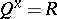and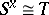as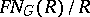-modules. The "non-blockwise" version of Alperin's weight conjecture simply asserts that the number of isomorphism types of simple-modules should equal the number of equivalence classes of weights of. The number of equivalence classes of weights of the formis just the number of isomorphism types of projective simple-modules, and the number of equivalence classes of weights of the formwith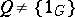is determined within-local subgroups.

To describe the "blockwise" version of the conjecture one has to assign weights to blocks. This is achieved by means of the Brauer homomorphism. When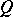is a-subgroup of, the projectionwith kernel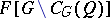restricts to an algebra homomorphism from the fixed-point subalgebra(under conjugation by) onto. Given a weight, one may viewas an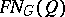-module. One assigns the weightto the blockif and only if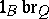does not annihilate. The "blockwise" version of Alperin's weight conjecture predicts that for each blockof,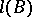should equal the number of equivalence classes of weights assigned to. Ifis of defect, this is evidently true. Ifis of positive defect, then the conjecture makes a prediction thatis-locally determined in a precise manner, since no weights of the form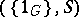are then assigned to.

Another interpretation of Alperin's weight conjecture has been given by R. Knörr and G.R. Robinson. Given a block, Brauer showed how to assign complex irreducible characters to, and the number of such characters assigned tois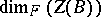, denoted.

Given a chainof strictly increasing-subgroups of, say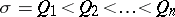, one sets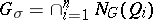, and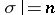. Then one sets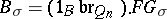, which is a sum of certain blocks of the group algebra. Then the blockwise version of Alperin's weight conjecture is equivalent to the assertion that wheneveris a block of positive defect offor some finite group, then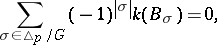where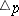denotes the collection of all such chains of-subgroups of(including the empty chain). Thus,(the contribution to the alternating sum from the empty chain) is predicted to be-locally controlled in a precise fashion.

J. Thévenaz has given a reformulation of Alperin's conjecture which is expressed in terms of equivariant-theory. E.C. Dade has continued the pattern of predictions of precise-local control of representation-theoretic invariants by making a series of conjectures expressing the number of irreducible characters of defect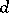assigned to a blockofas an alternating sum somewhat similar to that above. An irreducible characteris said to have defectfor the prime numberif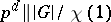. Dade's conjectures may be viewed as unifying and extending Alperin's weight conjecture and the Alperin–McKay conjecture. Furthermore, they are compatible with techniques of Clifford theory and offer the prospect of reducing these questions to questions about finite simple groups (cf. Simple finite group).